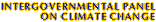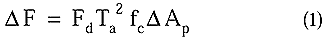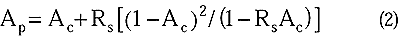Climate Change 2001: Working Group I: The Scientific BasisGet Javascript Other reports in this collection

### 5.4.5 Assessment of the Uncertainty in Indirect Forcing of the First Kind

Estimation of the uncertainty in the complete (i.e. the first and second indirect effects) radiative forcing is not currently feasible due to a lack of analytical relationships to treat the indirect forcing of the second kind. However, the indirect forcing of the first kind can be treated if we adopt a simple box-model approach such as those used in early assessments of indirect forcing (e.g., Charlson et al., 1992; Schwartz and Slingo, 1996).This evaluation of uncertainty is only illustrative both because of the box-model nature of the estimate and because our assessment of the uncertainty in the parameters is only first order. Nevertheless, it is useful to make such calculations since they can yield valuable information both on our current state of knowledge with regard to the indirect forcing and can help guide efforts to reduce uncertainty. Moreover they illustrate a rigorous method that could allow a more quantitative estimate of uncertainty than the methods followed in Chapter 6.

We adopt a functional relationship between sulphate concentrations and cloud droplet number concentration based on empirical relationships in order to render the calculations more tractable. Therefore, this analysis is only applicable to the Northern Hemisphere, since data from this region were used to derive the empirical relationship. The analysis is further restricted to the marine atmosphere and excludes any consideration of indirect forcing by biomass aerosols.

The indirect forcing of the first kind ( hereafter called simply the indirect forcing) can be expressed as:where Fd is the average downward flux at the top of the atmosphere, Ta is the atmospheric transmission above the cloud layer, fc is the fractional cloud cover of those clouds susceptible to aerosol modulation and DAp is the change in planetary albedo (equivalent here to the above cloud albedo) associated with an increase in the cloud droplet number concentration (CDNC). To take into account multiple reflections between the cloud layer and the surface, the expression of Liou (1980) is used with an assumption of no absorption within the cloud. This assumption is very reasonable for the bulk of the incoming radiation which will be scattered by cloud drops, i.e., subject to the indirect effect, but does restrict the radiation band to a range from 0.3 to 0.7 mm. Thus:where Ac is the cloud albedo and Rs is the albedo of the underlying surface. DAp is then calculated as the difference between this function evaluated for Ac, the background cloud albedo, and Ac', the anthropogenically perturbed albedo (note that primed quantities will always refer to anthropogenically perturbed values of the quantity). Cloud albedo is, in turn, evaluated using the relationship:which is an approximation of the two stream evaluation of cloud albedo for conservative scattering assuming an asymmetry parameter of 0.85 (Lacis and Hansen, 1974). Here, tc is the cloud optical depth, given by the expression of Twomey (1977):where h is the cloud layer thickness, LWC is the layer mean liquid-water content, Nd is the CDNC, and r is the density of water. To relate the CDNC to anthropogenic emissions, we use the empirical expression of Boucher and Lohmann (1995) which has the form:where SO42- is the mean concentration of sulphate aerosol at cloud base in µg m-3, and A and B are empirical constants. We adopt the values of A=115 and B=0.48 which are appropriate for marine air (Boucher and Lohmann, 1995).

Using the same procedures as with the assessment of uncertainties in the direct forcing (e.g. Section 5.4.2), we first determine the uncertainties in the most fundamental parameters. We then use Taylor expansions of the various equations given above to determine the uncertainty in the forcing associated with the central values of the parameters used in the calculations. Thus the uncertainty involves the uncertainties in the concentration of SO42- and in the empirical coefficients used to relate the concentration of SO42- to Nd. Moreover, the calculation of uncertainty necessarily involves an evaluation of Ac for both the background and anthropogenically perturbed values. These quantities, together with corresponding values for LWC and h, which are based on available observations, are then used to generate uncertainties in the cloud optical depth. This hierarchical evaluation proceeds upward from the uncertainty in the primary variables until the uncertainty in the forcing itself, together with the associated central value can be assessed. Such an uncertainty estimate is different in philosophy from that used in Chapter 6 which only assesses the range of estimates in the literature.

Continues on next page